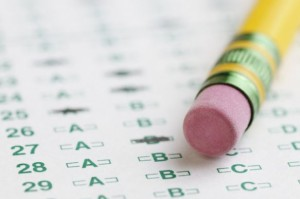## General Aptitude Test PhilippinesAptitude tests in Philippines are basically structured to evaluate the way people perform different tasks. The tests often involve administration of questions with multiple choices similar to an exam condition. Just as in exams, the questions are timed to typically allow 1 minute for each question. After the questions, the answers are marked under standardized methods and scores awarded after a comparison on how the others have completed the same tests. Aptitude tests may take many forms but the general aptitude is the most common.

In the General aptitude test Philippines, a combination of general questions similar to those asked while applying for a job or during exam admittance in schools is asked. While undertaking these tests, individuals are recommended to only use a pen and a paper and are supposed to be solved mentally without the use of a calculator. The general aptitude tests are grouped in different categories with the most common ones discussed below.

### Numeric Logic tests

Here one is provided with numerical questions that will require you to find missing numbers in a sequence. All of the questions and numbers usually have a normal, defined or a mathematical pattern that you will be required to identify. In order to answer the questions correctly, you must first identify the pattern after which you can proceed to complete calculations for the missing number. This kind of questions is widely used in innumerable areas including school admittance tests, intelligence tests as well as during employer’s aptitude testing.

### Numerical reasoningThe main essence of this type of test is to understand a person’s general numerical aptitude. The tests may take two forms including complex calculations that presented in a simple format or simple calculations that have been presented in a complex format. The tests are basically used in employer’s applicant screening with an aim of trying to rate the reasoning ability of the applicants.

### Data interpretation

Just as its name sounds, the tests aim at gauging one’s ability to extract correct data from various statistical methods of data representation. In order to get the correct answer, the data collected must be manipulated mathematically. In this type of tests, applicants are allowed to use pens, papers, and even calculators.

### Mathematical Knowledge tests

Mathematical Knowledge tests are mainly used in schools and are formulated to help gauge one’s knowledge in the general mathematics principles and rules. In most of the cases, the tests involve solving quadratic equations, finding out surface areas and volumes among many others. Calculators, pen and a paper are a key recommendation in these tests.

Rely on V-Psyche for general aptitude tests in Philippines!